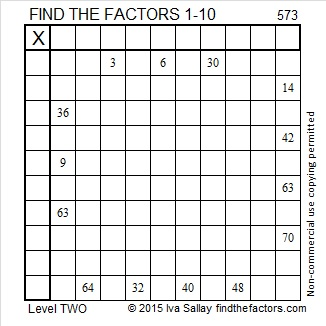# 573 and Level 2

573 is made from three consecutive odd numbers so it is divisible by 3. The number in the middle, 5, is not divisible by 3, so 573 is not divisible by 9.

573 squared is 328,329, a 6 digit number that looks like two consecutive numbers! Thank you OEIS.org for that interesting fact.Print the puzzles or type the solution on this excel file: 10 Factors 2015-08-03

—————————————————————————————————

• 573 is a composite number.
• Prime factorization: 573 = 3 x 191
• The exponents in the prime factorization are 1 and 1. Adding one to each and multiplying we get (1 + 1)(1 + 1) = 2 x 2 = 4. Therefore 573 has exactly 4 factors.
• Factors of 573: 1, 3, 191, 573
• Factor pairs: 573 = 1 x 573 or 3 x 191
• 573 has no square factors that allow its square root to be simplified. √573 ≈ 23.9374—————————————————————————————————This site uses Akismet to reduce spam. Learn how your comment data is processed.